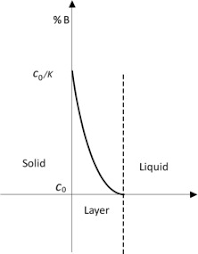## How to Calculate and Solve for Thickness of Solidifying Metals | Solidification of MetalsThe image above represents thickness of solidifying metals.

To compute for thickness of solidifying metals, six essential parameters are needed and these parameters are Melting Temperature of Metal (Tm), Initial Mould Temperature (To), Heat Diffusivity (α), Time (t), Density (ρ’) and Latent Heat of Fusion (Hf).

The formula for calculating thickness of solidifying metals:

M = 2(Tm – To)√(α)√(t) / √(π)ρ’Hf

Where:

M = Thickness of Solidifying Metal
Tm = Melting Temperature of Metal
To = Initial Mould Temperature
α = Heat Diffusivity
t = Time
ρ = Density
Hf = Latent Heat of Fusion

Let’s solve an example;
Find the thickness of solidifying metal when the melting temperature of metal is 4, the initial mould temperature is 8, the heat diffusivity is 2, the time is 6, the density is 3 and the latent heat of fusion is 7.

This implies that;

Tm = Melting Temperature of Metal = 4
To = Initial Mould Temperature = 8
α = Heat Diffusivity = 2
t = Time = 6
ρ = Density = 3
Hf = Latent Heat of Fusion = 7

M = 2(Tm – To)√(α)√(t) / √(π)ρ’Hf
M = 2(4 – 8)√(2)√(6) / √(π)(3)(7)
M = 2(-4)(1.414)(2.449) / (1.772)(3)(7)
M = -27.712 / 37.22
M = -0.744

Therefore, the thickness of solidifying metal is -0.744 m.